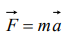## Laws of Motion Questions and Answers Part-1

1. Newton's first law of motion describes the following
a) Energy
b) Work
c) Inertia
d) Moment of inertia

Explanation: Newton’s first law of motion defines the inertia of body. It states that every body has a tendency to remain in its state (either rest or motion) due to its inertia

2. A person sitting in an open car moving at constant velocity throws a ball vertically up into air. The ball falls
a) Outside the car
b) In the car ahead of the person
c) In the car to the side of the person
d) Exactly in the hand which threw it up

Explanation: Horizontal velocity of ball and person are same so both will cover equal horizontal distance in a given interval of time and after following the parabolic path the ball falls exactly in the hand which threw it up

3. A bird weighs 2 kg and is inside a closed cage of 1 kg. If it starts flying, then what is the weight of the bird and cage assembly
a) 1.5 kg
b) 2.5 kg
c) 3 kg
d) 4 kg

Explanation: When the bird flies, it pushes air down to balance its weight. So the weight of the bird and closed cage assembly remains unchanged

4. A particle is moving with a constant speed along a straight line path. A force is not required to
a) Increase its speed
b) Decrease the momentum
c) Change the direction
d) Keep it moving with uniform velocity

Explanation: Particle will move with uniform velocity due to inertia.

5. When a bus suddenly takes a turn, the passengers are thrown outwards because of
a) Inertia of motion
b) Acceleration of motion
c) Speed of motion
d) Both (b) and (c)

Explanation: Inertia of motion

6. A rocket is propelled by a gas which is initially at a temperature of 4000 K. The temperature of the gas falls to 1000 K as it leaves the exhaust nozzle. The gas which will acquire the largest momentum while leaving the nozzle, is
a) Hydrogen
b) Helium
c) Nitrogen
d) Argon

Explanation: Heavier gas will acquire largest momentum i.e. Argon

7. If a bullet of mass 5 gm moving with velocity 100 m /sec, penetrates the wooden block upto 6 cm. Then the average force imposed by the bullet on the block is
a) 8300 N
b) 417 N
c) 830 N
d) Zero

Explanation:8. Newton's second law gives the measure of
a) Acceleration
b) Force
c) Momentum
d) Angular momentum

Explanation:9. A force of 100 dynes acts on mass of 5 gm for 10 sec. The velocity produced is
a) 2 cm/sec
b) 20 cm/sec
c) 200 cm/sec
d) 2000 cm/sec